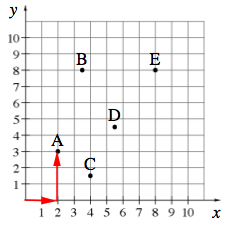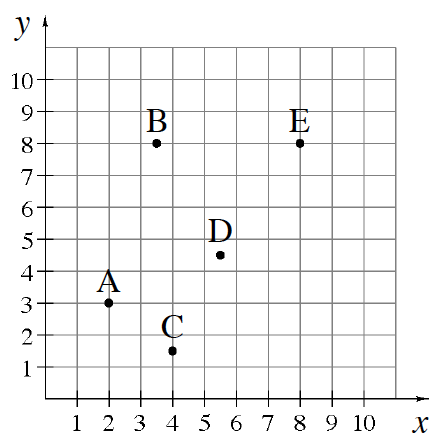### Home > ACC6 > Chapter 6 Unit 6 > Lesson CC1: 6.1.2 > Problem6-25

6-25.

Write the points on the graph at right as ordered pairs.

Point B is $3.5$ units to the right and $8$ units up. Therefore, point B is at $(3.5, ?)$.
Point C is $4$ units to the right and $1.5$ units up. Therefore, point C is at $(?, 1.5)$.
Point D is $5.5$ units to the right and $4.5$ units up.
Write the ordered pair of point E by using the information given for the other points.From the origin, point A is $2$ units to the right and $3$ units up. Therefore, point A is at $(2, 3)$.